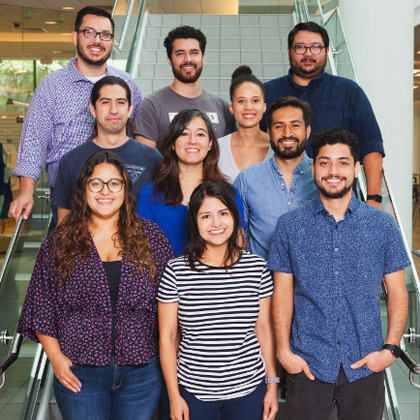# Pytorch FaceNet MTCNN model for facial features

I am using MTCNN to detect face in an image, FACENET to extract and save features from each detected face and OpenCV Gaussian Blur filter to mask the detected faces.
My end goal is to find the target face in the masked image by comparing saved facial features(or any other method) and unmask target face only. But the features detected by FaceNet for the face in the full image and a cropped face are different.Here is how my code looks:

``````def face_and_features(img):
boxes, _ = mtcnn.detect(img)
print(boxes)

for i, box in enumerate(boxes):
a,b,c,d = box
x1,y1,x2,y2 = int(a), int(b), int(c), int(d)
face = img[y1:y2, x1:x2]
cv2.imwrite(f"face{i}.jpg",face)

face = cv2.resize(face, (160, 160))
face = face.transpose((2, 0, 1))
face = torch.from_numpy(face).float()
face = face.unsqueeze(0)

features = facenet(face)

filename = "face_{}.npy".format(i)
np.save(filename, features.detach().numpy())
with open("bounding_boxes.txt", "a") as f:
f.write("{}, {}, {}, {}, {}, {}, {} \n".format(x1, y1, x2, y2, filename, datetime.datetime.now(), frame_number))
return features

filename = "bounding_boxes.txt"
with open(filename,'r') as file:
for line in file:
x,y,w,h,f_name,time, f_no = line.split(",")
x,y,w,h = int(x), int(y), int(w), int(h)
masked_img[y:h, x:w] = cv2.GaussianBlur(roi_color, (51,51), 0)

def compare_features(target_face, saved_features):
target_face = cv2.resize(target_face, (160, 160))
target_face = target_face.transpose((2, 0, 1))
target_face = torch.from_numpy(target_face).float()
target_face = target_face.unsqueeze(0)

target_features = facenet(target_face)
filename = "target_face.npy"
np.save(filename, target_features.detach().numpy())